# Matrix

For the 1999 film, see The Matrix.

A matrix (pl.: "matrices," Latin origin) is a complex ordering, in deliberate fashion, of numerals. In mathematics, a "matrix" is a regular grid of numbers, which may be manipulated and solved through intermediate-level algebra. Matrix algebra is usually taught in sophomore high school level mathematics.

More formally, a matrix is an example of a rank-2 tensor.

Alternately, a matrix may also be a complex ordering of a group of equivalent objects, especially where the order is imposed to gain incidental benefit from the synergy of the networked objects.

## Mathematics

In mathematics, matrices can be manipulated in a variety of ways, including addition and multiplication.

To add two matrices, one would add their respective elements. For example: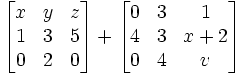would equal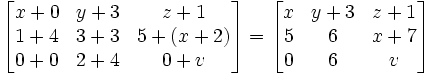### Multiplication of matrices

To multiply two matrices, use the rule for finding the product of two matrices:However, not every pair of matrices can be multiplied. In order for matrices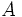andto be compatible for multiplication, the number of columns inmust equal the number of rows in. Ifis an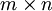matrix andis anmatrix, the product matrix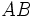will have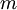rows and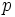columns.

Matrix multiplication is associative. However, matrix multiplication is not commutative. That is, it is possible for.

The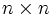identity matrix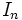satisfies the property:for allmatrices.

Moreover, every square matrixwith nonzero determinant has an inverse matrixsuch that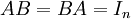This means that for every positive integer, the set of allmatrices with nonzero determinant form a group under matrix multiplication. This group is known as the general linear group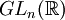.